# Two machines are used for filling plastic bottles with a net volume of 16.0 ounces. The...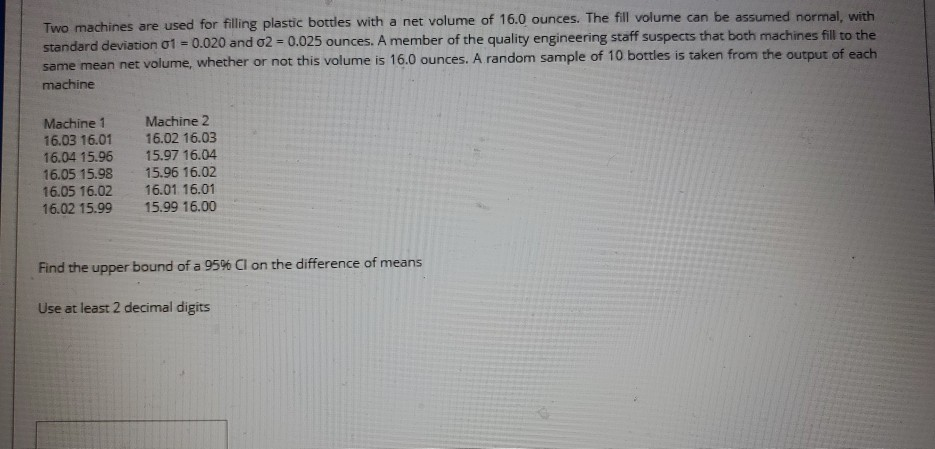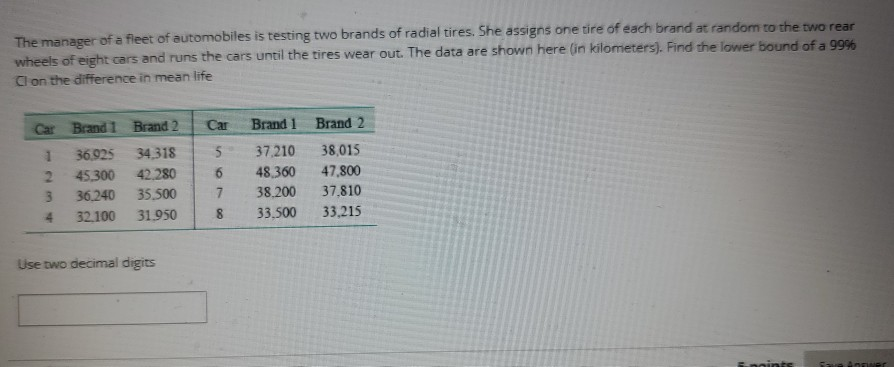Two machines are used for filling plastic bottles with a net volume of 16.0 ounces. The fill volume can be assumed normal, with standard deviation o1 = 0.020 and 02 = 0.025 ounces. A member of the quality engineering staff suspects that both machines fill to the same mean net volume, whether or not this volume is 16.0 ounces. A random sample of 10 bottles is taken from the output of each machine Machine 1 16.03 16.01 16.04 15.96 16.05 15.98 16.05 16.02 16.02 15.99 Machine 2 16.02 16.03 15.97 16.04 15.96 16.02 16.01. 16.01 15.99 16.00 Find the upper bound of a 95% Cl on the difference of means Use at least 2 decimal digits
The manager of a fleet of automobiles is testing two brands of radial tires. She assigns one tire of each brand at random to the two rear wheels of eight cars and runs the cars until the tires wear out. The data are shown here (in kilometers). Find the lower bound of a 99% Cl on the difference in mean life Car Brand 2 Car Brand 1 Brand 2 36.925 34 318 2 45.300 42.280 36.240 35.500 4 32.100 31.950 5 6 Brand 1 37.210 48,360 38.200 33.500 38,015 47,800 37.810 33.215 8 Use two decimal digits

1)

 X1 bar 16.015 (AVERAGE()) X2 bar 16.005 S1 0.02 (GIVEN) S2 0.025 n1 10 n2 10
 95%CI α = 0.05 Zc 1.959964 +/-   NORM.S.INV(α/2) ME 0.019843 Zc*SQRT(S1^2/n+S2^2/n) Upper 0.029843 (X1 bar-X2 bar) + Zc*SQRT(S1^2/n+S2^2/n) Lower -0.00984 (X1 bar-X2 bar) - Zc*SQRT(S1^2/n+S2^2/n)

Upper bound = 0.03

2)

 Sample1 Sample 2 Diff 36925 34318 2607 45300 42280 3020 36240 35500 740 32100 31950 150 37210 38015 -805 48360 47800 560 38200 37810 390 33500 33215 285 Mean Dbar 868.375 Sd Sd 1290.033 n 8
 99% CI α = 0.01 tc 3.499483 T.INV.2T(alpha,df) ME 1596.099 tc*(Sd/SQRT(n)) Upper 2464.474 D bar + tc*(Sd/SQRT(n)) Lower -727.724 D bar - tc*(Sd/SQRT(n))

Lower bound = -727.72

##### Add Answer of: Two machines are used for filling plastic bottles with a net volume of 16.0 ounces. The...
Similar Homework Help Questions
• ### The manager of a fleet of automobiles is testing two brands of radial tires. She assigns...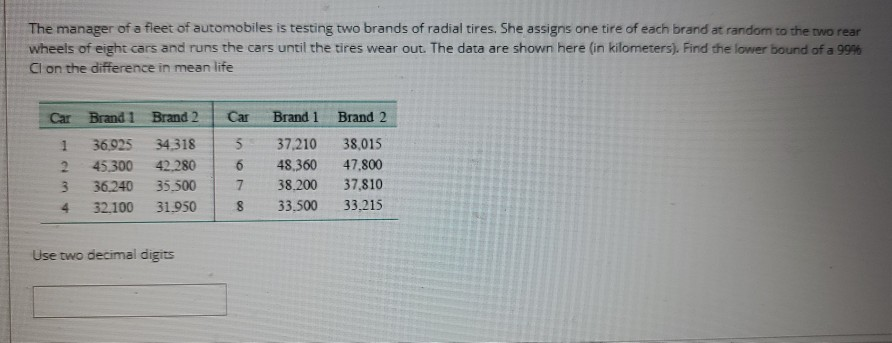The manager of a fleet of automobiles is testing two brands of radial tires. She assigns one tire of each brand at random to the two rear wheels of eight cars and runs the cars until the tires wear out. The data are shown here (in kilometers). Find the lower bound of a 999 Cl on the difference in mean life Car Brand 1 Brand 2 Car Brand 1 Brand 2 1 36,925 34 318 45.300 42.280 3 36.240 35.500...

• ### 5. Two machines are used for filling plastic bottles to a net volume of 16.0 ounces....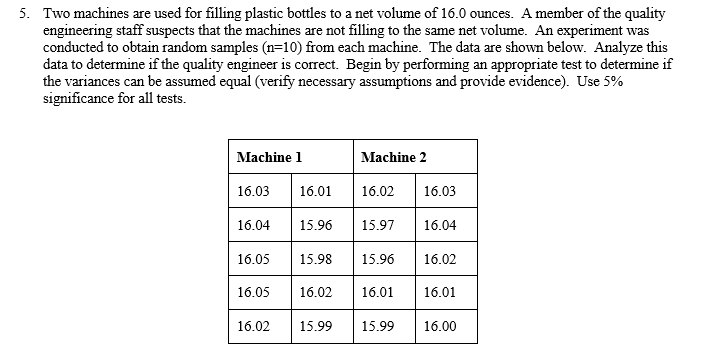5. Two machines are used for filling plastic bottles to a net volume of 16.0 ounces. A member of the quality engineering staff suspects that the machines are not filling to the same net volume. An experiment was conducted to obtain random samples (n=10) from each machine. The data are shown below. Analyze this data to determine if the quality engineer is correct. Begin by performing an appropriate test to determine if the variances can be assumed equal (verify necessary...

• ### and with minitab please. 5. Two machines are used for filling plastic bottles to a net...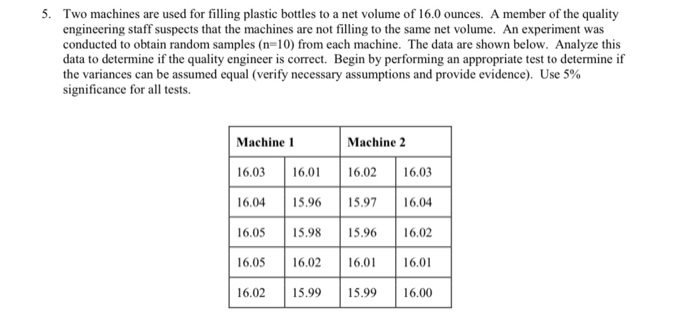and with minitab please. 5. Two machines are used for filling plastic bottles to a net volume of 16.0 ounces. A member of the quality engineering staff suspects that the machines are not filling to the same net volume. An experiment was conducted to obtain random samples (n=10) from each machine. The data are shown below. Analyze this data to determine if the quality engineer is correct. Begin by performing an appropriate test to determine if the variances can be...

• ### 2side Hypothesis Testing_03 (two independent sam machines are used for filling plastic battle with a new volume of...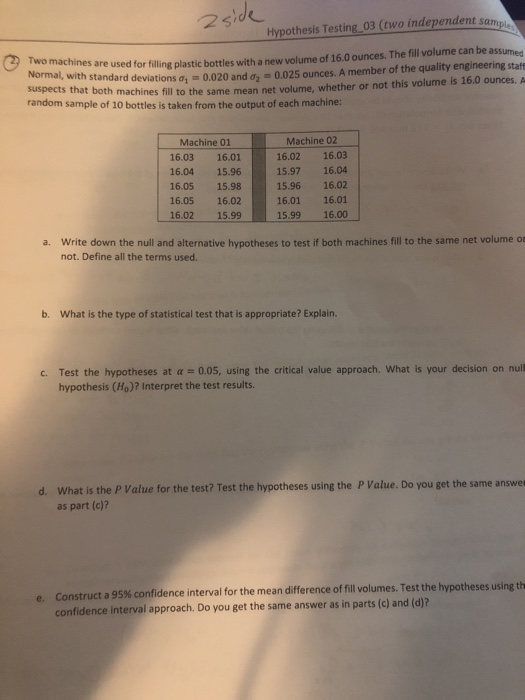2side Hypothesis Testing_03 (two independent sam machines are used for filling plastic battle with a new volume of 16.0 ounces. The fill volume can be assumed was with standard deviations d. 0.020 and 0.025 ounces. A member of the quality engineering staff that both machines fill to the same mean net volume, whether or not this volume is 16.0 ounces. random sample of 10 bottles is taken from the output of each machine: Machine 01 16.03 16.01 16.04 15.96 16.05...

• ### The melting points of two alloys used in formulating solder were investigated by melting 21 samples...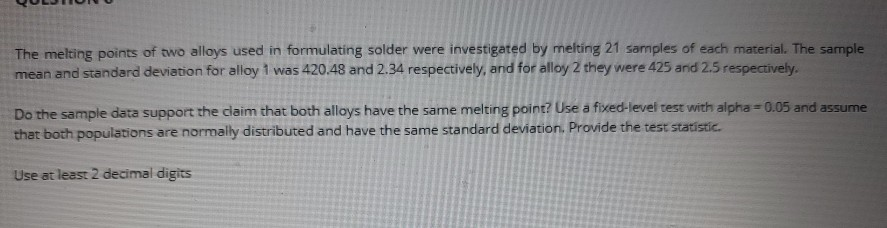The melting points of two alloys used in formulating solder were investigated by melting 21 samples of each material. The sample mean and standard deviation for alloy 1 was 420.48 and 2.34 respectively, and for alloy 2 they were 425 and 2.5 respectively. Do the sample data support the claim that both alloys have the same melting point? Use a fixed-level test with alpha=0.05 and assume that both populations are normally distributed and have the same standard deviation. Provide the...

• ### Statistics 12

Two machines are used for filling plastic bottles with a netvolume of 16.0 ounces. The filling processes can be assumed tobe normal, with standard deviationof1 = 0.015 and2 = 0.018. The quality engineeringdepartment suspects that both machines fill to thesame net volume,whether or not this volume is 16.0 ounces. An experiment isperformed by taking a random sample from the output of eachmachine.Machine 1Machine 216.0316.0116.0216.0316.0415.9615.9716.0416.0515.9815.9616.0216.0516.0216.0116.0116.0215.9915.9916.00(a) State the hypotheses that should be tested in thisexperiment (b) Test thesehypotheses using =0.05. What are...

• ### S: 10-2 Q: 10-4

Two machines are used for filling plasticbottles with a net volume of 16.0 ounces.The fill volumecanbe assumed normal, with standard deviationσ1= .02 andσ2= .025 ounces.A member of the quality engineeringstaff suspects that both machines fill to the same mean net volume,wether or notthis volume is 16 ounces. A random sample of 10bottles is taken from the output of each machine.Machine 1Machine 216.0316.0116.0216.0316.0415.9615.9716.0416.0515.9815.9616.0216.0516.0216.0116.0116.00215.9915.9916(a) Do you think the engineer is correct? Useα= 0.05.What is the P-Value for this test?(b) Calculate a 95%...

• ### 3. Two machines are used for filling plastic bottles with a net volume of 16.0 ounces....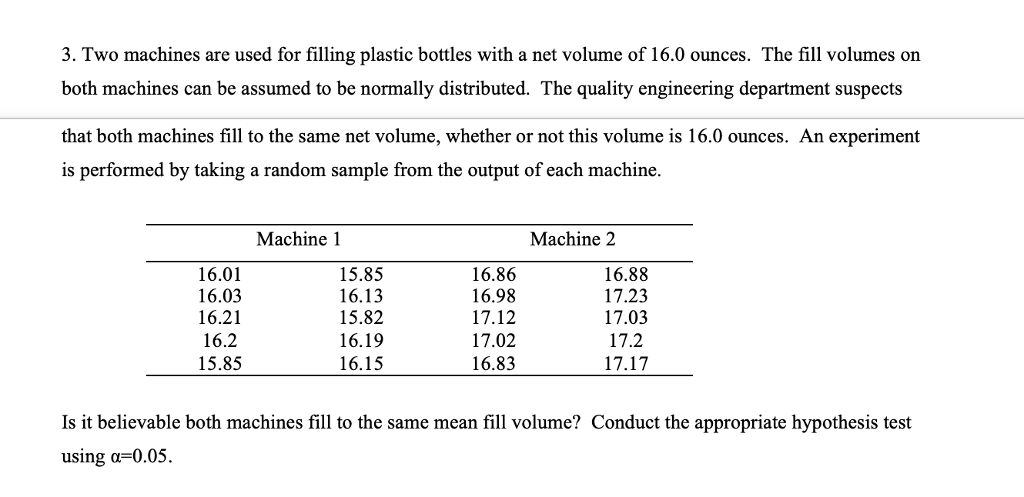3. Two machines are used for filling plastic bottles with a net volume of 16.0 ounces. The fill volumes on both machines can be assumed to be normally distributed. The quality engineering department suspects that both machines fill to the same net volume, whether or not this volume is 16.0 ounces. An experiment is performed by taking a random sample from the output of each machine Machine 1 Machine 2 16.01 16.03 16.21 16.2 15.85 15.85 16.13 15.82 16.19 16.15...

• ### A quality-control engineer wants to find out whether or not a new machine that fills bottles...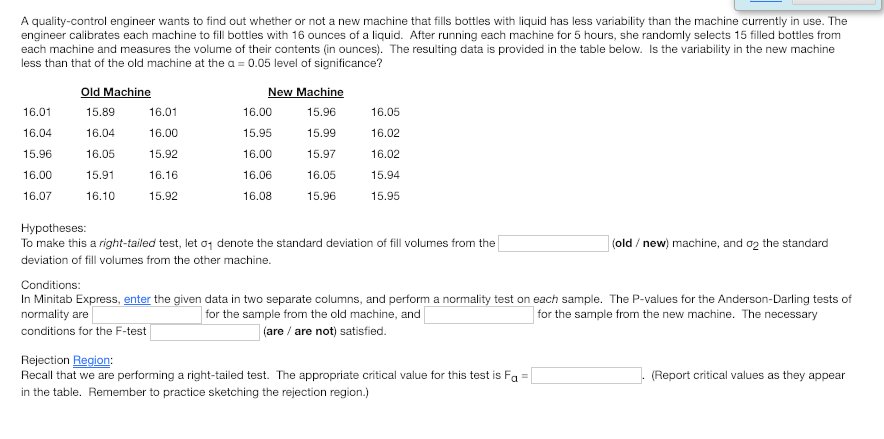A quality-control engineer wants to find out whether or not a new machine that fills bottles with liquid has less variability than the machine currently in use. The engineer calibrates each machine to fill bottles with 16 ounces of a liquid. After running each machine for 5 hours, she randomly selects 15 filled bottles from each machine and measures the volume of their contents (in ounces). The resulting data is provided in the table below. Is the variability in the...

• ### . Two machines are used to ﬁll plastic bottles with dish-washing detergent. The standard deviations of ﬁll volume are kn...

. Two machines are used to ﬁll plastic bottles with dish-washing detergent. The standard deviations of ﬁll volume are known to be σ1 = 0.10 and σ2 = 0.15 ﬂuid ounces for the two machines, respectively. Two random samples of n1 = 12 bottles from machine 1 and n2 = 10 bottles from machine 2 are selected, and the sample mean ﬁll volumes are ¯ x1 = 30.61 and ¯ x2 = 30.34 ﬂuid ounces. Assume normality and independence. (a)...

Free Homework App